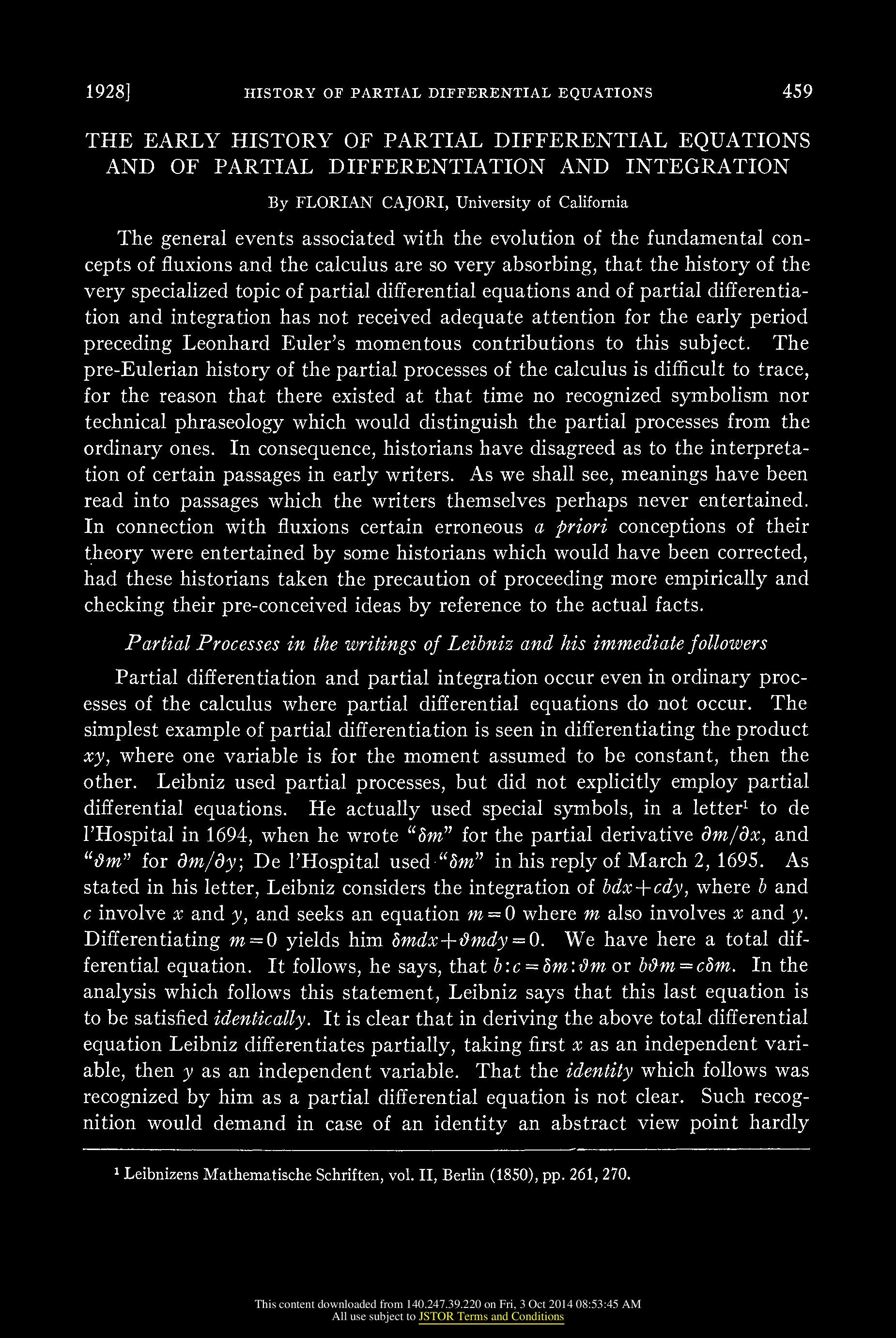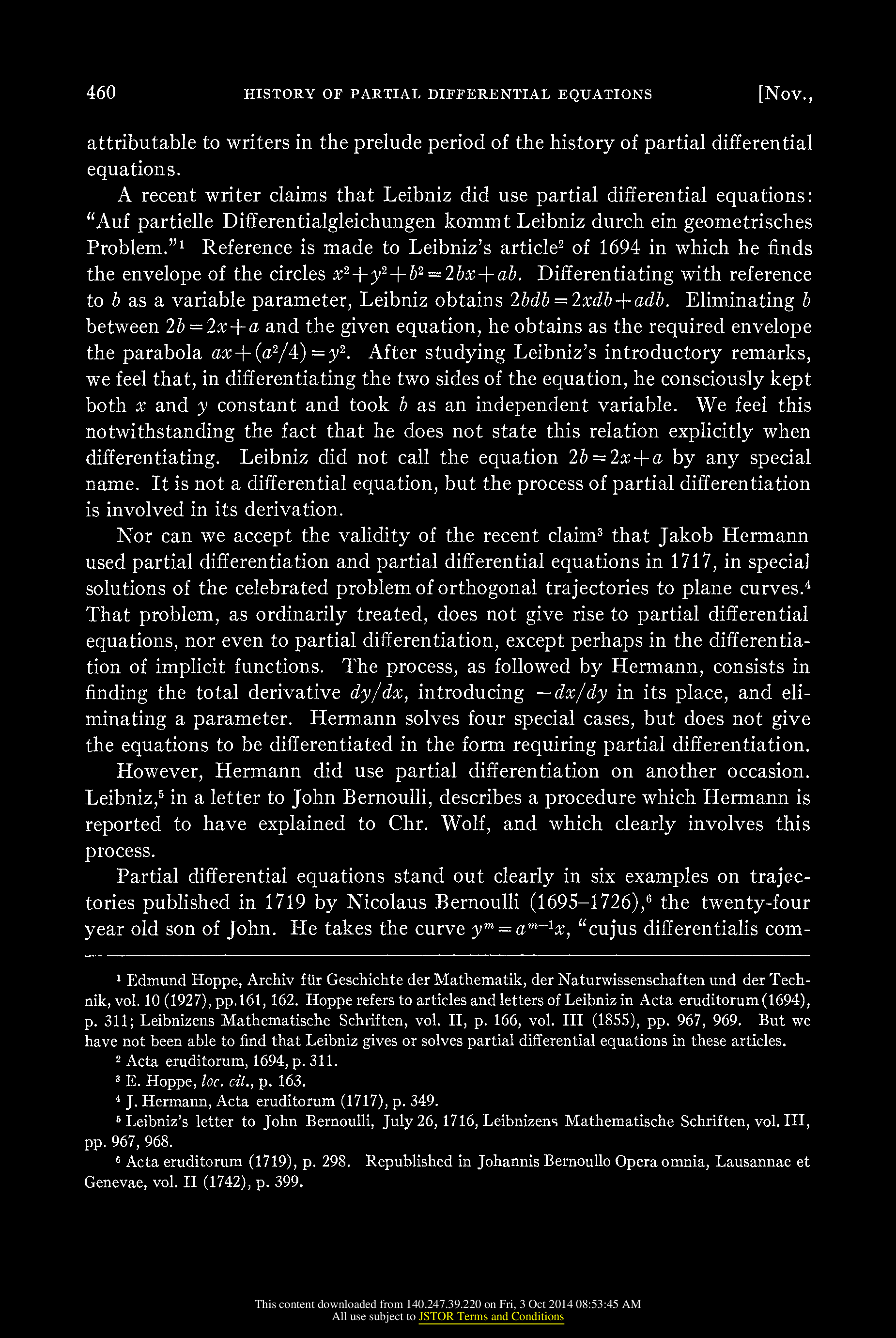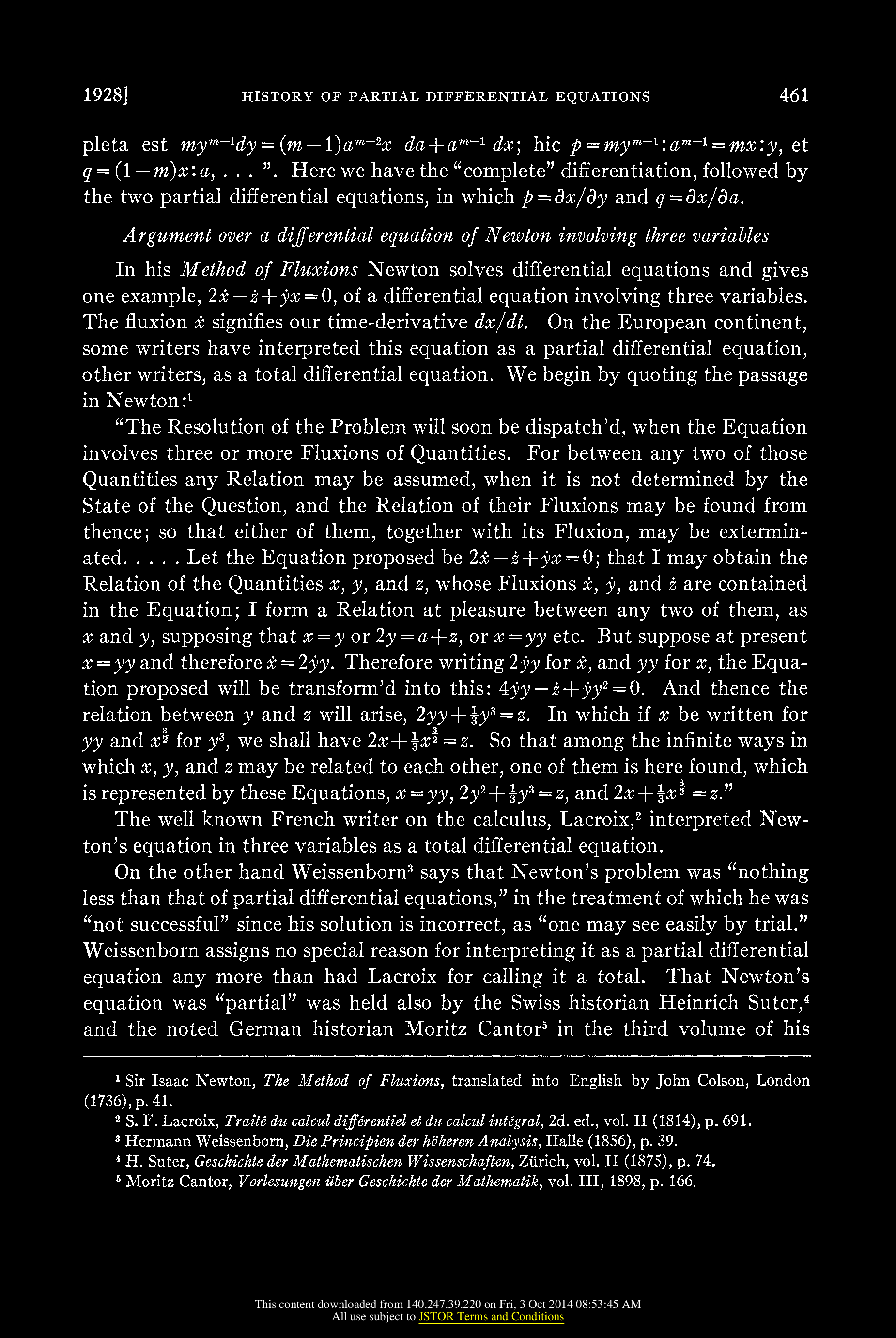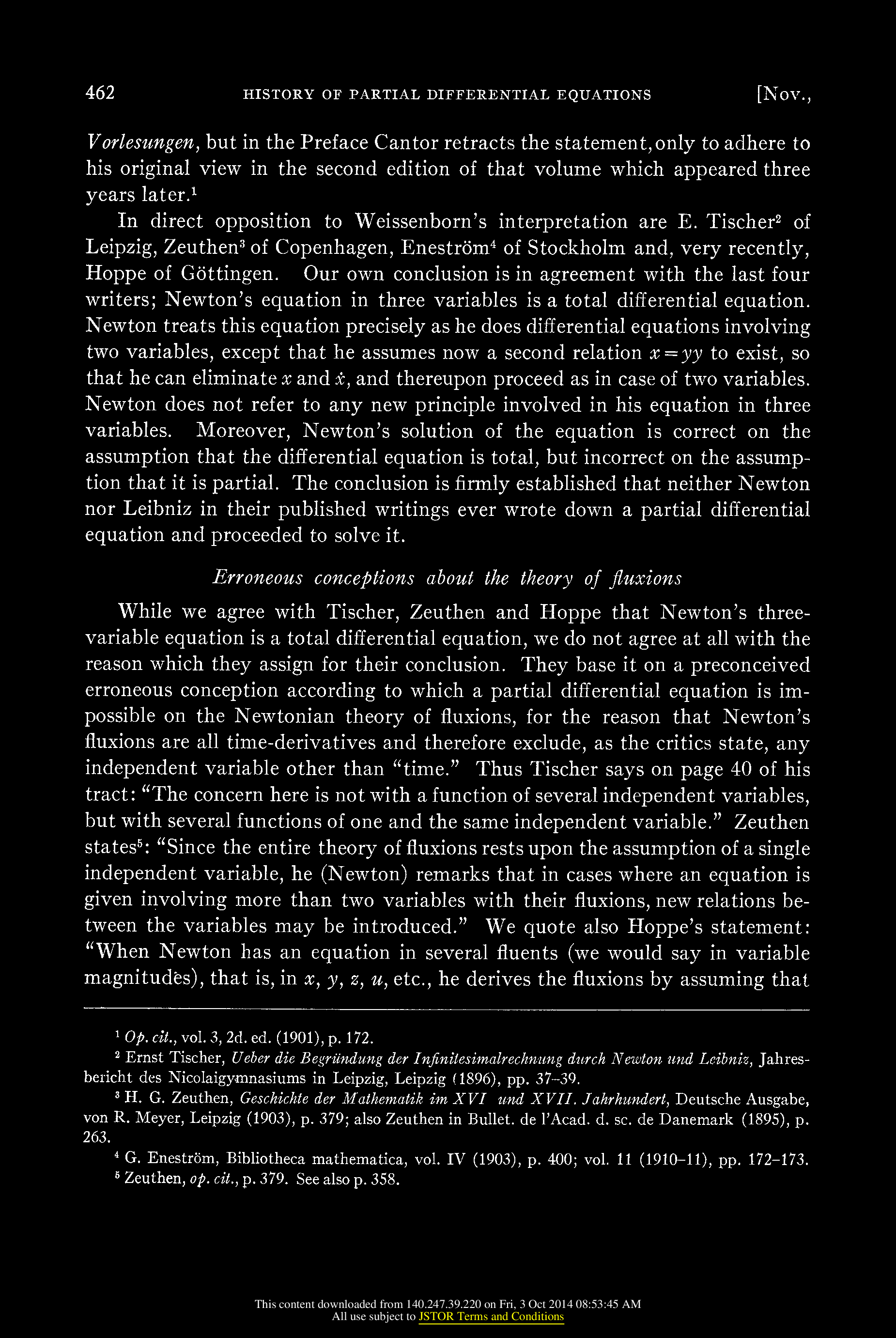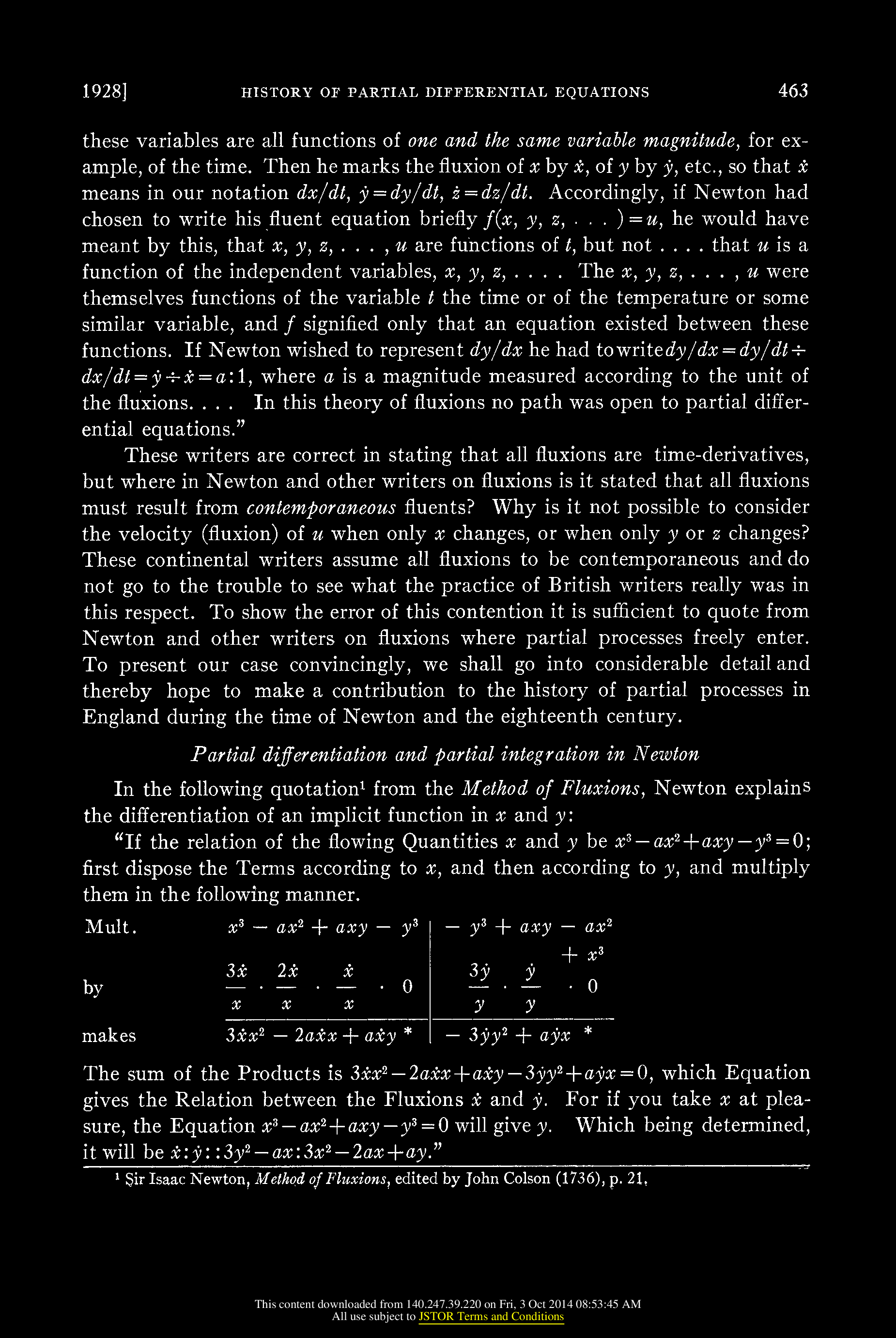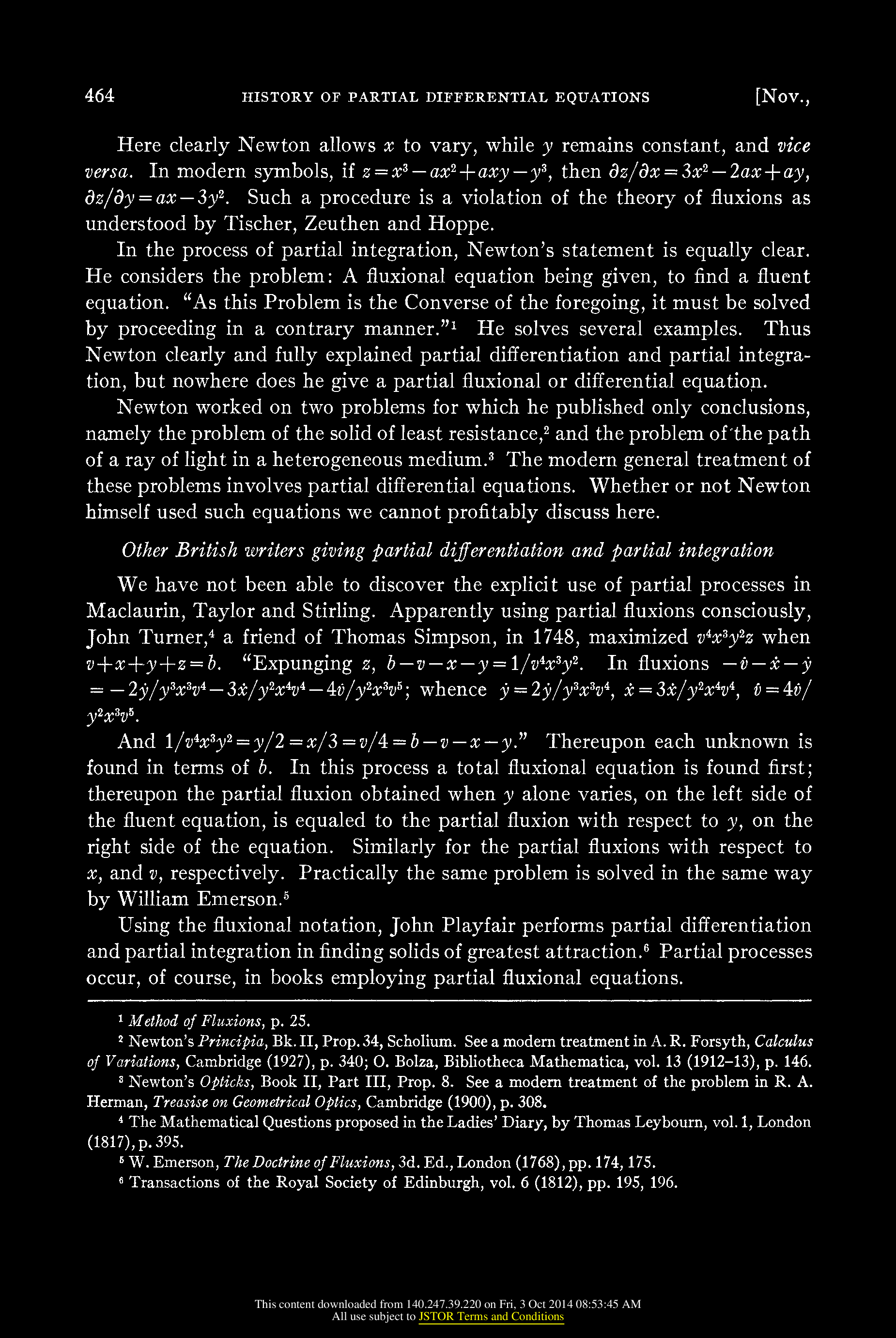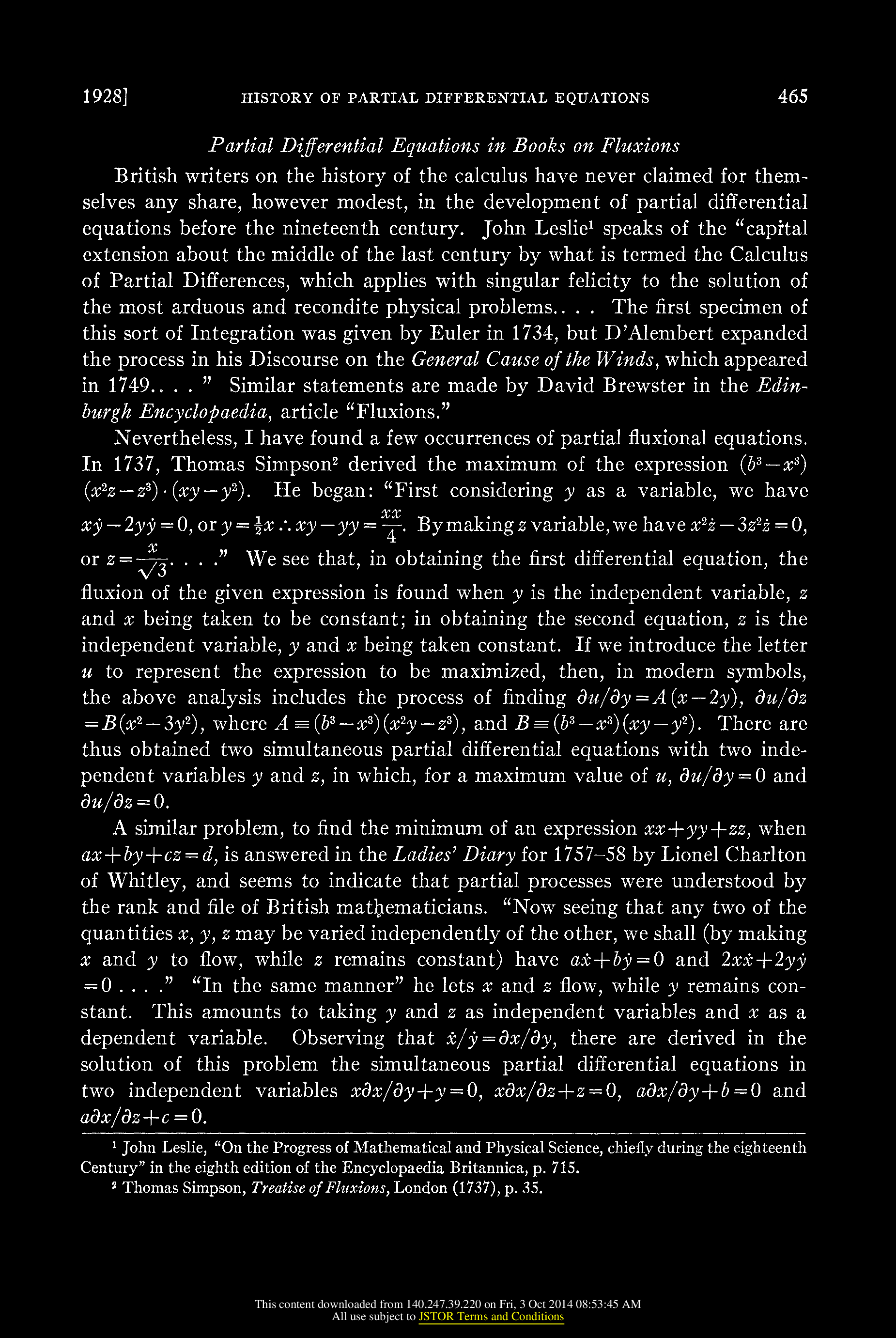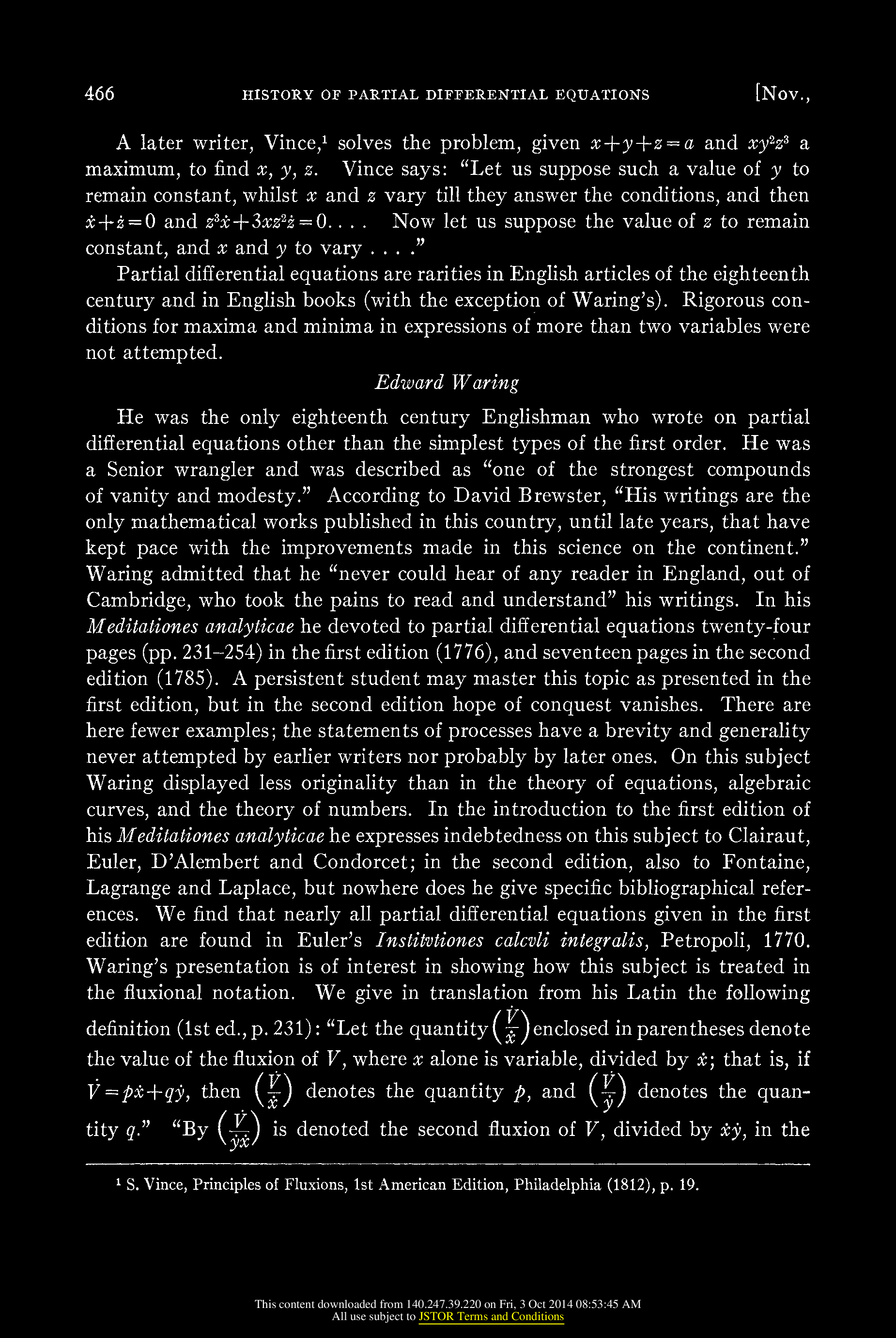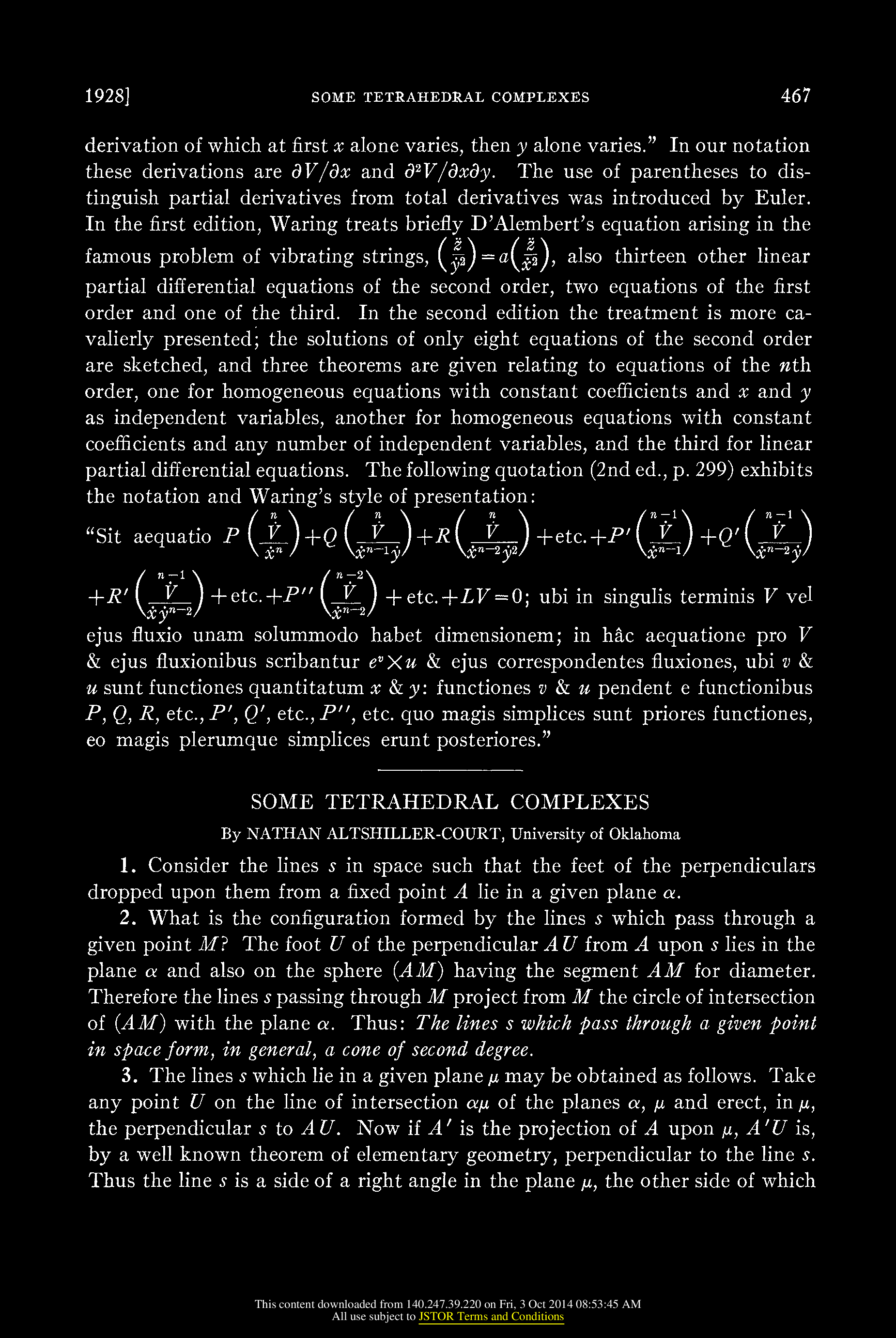An article of Cajori [PDF] "The early history of partial differential equations and of partial differentiation and integration" from the American Mathematical Monthly Vol 35, p.459-467, 1928 gives some historical pointers about the origin of calculus. It seems that Leibniz already used partial differentiation even so he did not consider partial differential equations. The notation of Leibniz stayed. As the article states, the question whether Leibniz used partial differential equations already is under dispute. Cajori argues that it was Nicolaus Bernoulli who used PDEs first in 1719 and not Leibniz in 1717.

Newton had a strange way to write about derivatives. His Method of Fluxions solved ordinary differential equations. Also the question whether Newton used partial differential equations already is under dispute. Cajori argues that Newton did not use partial differential equations.

"The conclusion is firmly established that neither Newton nor Leibniz in their published writings ever wrote down a partial differential equation and proceeded to solve it."

Of course this assertion of Cajori assumes (as we do in our courses) that an ordinary differential equation is not a partial differential equation even so one could take the point of view that ordinary differential equations form a subset of partial differential equations, equations which only use the derivative with respect to one variable.